# Progressive Data Hiding in Integer Wavelet Transform of Electrocardiogram by Using Simple Decision Rule and Coefficient Calibration

Progressive Data Hiding in Integer Wavelet Transform of Electrocardiogram by Using Simple Decision Rule and Coefficient Calibration
National Penghu University of Science and Technology, 300, Liu-Ho Rd., Magong, Penghu 880, Taiwan

National Yunlin University of Science and Technology, 123 University Rd., Section 3, Douliou, Yunlin 64002, Taiwan

Corresponding Author Email:
chingyu@gms.npu.edu.tw
Page:
11-20
|
DOI:
https://doi.org/10.18280/ria.340102
3 November 2019
|
Accepted:
14 January 2020
|
Published:
29 February 2020
| Citation

OPEN ACCESS

Abstract:

Based on one dimensional (1D) integer wavelet transform (IWT) domain, patient’s data can be effectively embedded in electrocardiogram (ECG) via the proposed criterion and coefficient alignment. Multiple data bits can be sequentially hidden in host bundles (with different lengths) of the low and high subbands of IWT coefficients. Simulations indicated that the average signal-to-noise ratio (SNR) and the payload of the proposed method are superior to those of existing techniques. Additionally, the proposed method exhibited a robustness that has rarely been observed in conventional ECG steganography. Our method is capable of resisting attacks such as cropping, inversion, scaling, translation, truncation, and Gaussian noise addition. Because bit embedding and extraction procedures are quite simple, our method can be applied in portable biometric devices.

Keywords:

data hiding, ECG steganography, 1D IWT progressive bit embedding/extraction, coefficient alignment

1. Introduction

Due to the rapid evolution and growth of networking technology, the ubiquitous deployment of intelligent computing and automated devices, and various applications with user-friendly interfaces, people have become able to surf the Internet economically by using computing (or smart portable) devices. However, sensitive messages (or private data) are vulnerable to interception, copying, and tampering during transmission between a sender and an intended receiver. Encryption/decryption systems are commonly used to solve these problems. However, to reduce the cost and functional limitations of portable devices, various researchers have reported data hiding techniques as a solution. Recently, data hiding has played a crucial role in protecting secret data in multimedia files, such as documents, images, and videos [1-5]. In addition, several researchers have presented data hiding for biomedical signals such as an electrocardiogram (ECG) to secure personal sensitive information, including patients’ diagnoses [6-16].

In the context of the Fourier transform and spread spectrum approaches, Kozat et al.  proposed robust watermarking and fragile watermarking techniques for electrocardiograms (ECGs) to protect private data and the ownership of metadata. Simulations indicated that the robust watermarking technique exhibited effectiveness against manipulation, whereas the fragile watermarking technique effectively detected alteration. However, the payload size was <60 bits, and the resultant SNR was approximately 40 dB. To protect patients’ confidential information in point-of-care systems, Ibaida and Khalil  presented an efficient ECG steganography method that used encryption, scrambling, and wavelet packets. Simulations revealed that the perceived quality of marked ECGs was favorable with a low distortion rate of 1%. Chen et al.  reported three different kinds of ECG watermarking techniques to hide patients’ data in discrete wavelet transforms (DWTs), discrete cosine transforms, and discrete Fourier transforms. The average execution time was approximately 1.60 s and the size of the payload was only 32 bits; both of these problems may limit the usage of the method. On the basis of DWT and singular value decomposition, Jero et al.  proposed a noteworthy ECG steganography method to secure information about patients. Experimental results indicated that the bit error rate (BER) of their method was <0.6% because the high-high subband of DWT was used for hiding the secret information. However, their proposed method provided a payload size of 4,489 bits. By using curvet transform and quantization techniques, Jero and Ramu  developed a simple ECG steganography for data security. Simulations indicated that the percentage residual difference (PRD) and BER of the method were 0.110 and 31.84%, respectively; the size of the payload was 4,016 bits.

Based on the integer wavelet transform (IWT) domain and a coefficient adjustment technique, Yang and Lin  embedded data bits in the low and high subbands of IWT coefficients. Simulations confirmed that the average SNR introduced using the proposed method was approximately 42 dB with the payload of size 15,000 bits and a certain degree of robustness. Yang and Wang  employed a smart coefficient alignment technique and designed an effective ECG steganography for hiding patients’ information. The technique has two approaches: method A and method B. Experimental demonstrations indicated that method A created a high SNR value of approximately 55 dB with a payload size of 7,500 bits, whereas method B generated an SNR value of 44 dB with a large payload size of approximately 15,000 bits. By using DWT, singular value decomposition (SVD), and continuous ant colony optimization techniques, Jero et al.  embedded patient data in an ECG host. First, DWT decomposed a two dimensional ECG matrix into frequency subbands; and then, data bits were effectively hidden in the selected subbands by using SVD and quantization techniques. Experiments indicated that the resultant PRD was 0.015; the payload size was approximately 18,000 bits.

To obtain efficient wireless transmission, Pandey et al.  embedded sensitive data regarding patients in ECG signals by combining orthogonal frequency division multiplexing, chaotic map, and sample value difference methods. The average SNR of their method was 52 dB (or PRD = 0.260) with a payload size of 21,504 bits. To obtain a high-capacity ECG steganography, Yang and Wang  concealed patients’ diagnoses in biometric signals by embedding two data bits in a host bundle of the ECG. The payload size was 20,000 bits when the size of the host bundle was 3. Additionally, the average SNR of their method was approximately 45 dB. Instead of using auxiliary information, Yang and Wang  used the absolute-value-decision policy effectively promoting the SNR performance of the study . Furthermore, the number of input bits can be designed on-demand by using host bundles of various sizes. Experimental results indicated that the average SNR of their method was larger than that of the study  by approximately 3.54 dB.

In the aforementioned studies, the SNR, PRD, or resultant payload was not sufficiently favorable. In this study, we proposed an efficient ECG steganography method with the merits of high hiding capacity and perceived quality. Additionally, our method provided robust performance, which has rarely been observed in conventional ECG steganography methods. The remainder of this paper is organized as follows. Sec. 2 presents the procedures of bit embedding and bit extraction of our proposed method. Sec. 3 provides the simulation results. Sec. 4 presents conclusion of this study.

2. Proposed Method

To obtain a high hiding capacity with low computation time, and favorable perceived quality with robust performance, we embedded data bits in the IWT domain . First, an input ECG host was decomposed into low subband coefficients (IL) and high subband coefficients (IH) by using level 1 (L1) one dimensional (1D) IWT. Then, predetermined criteria for bit embedding and bit extraction were employed to conceal secret information in the IL and IH subbands, respectively. However, to obtain high perceived quality, data bits can be embedded only in the IH subband of IWT coefficients with host bundle sizes of <4. Although a multilevel IWT decomposition can be employed in our method to further promote robustness with approximate payload capability and similar perceptual quality, the cost of computation time might be an issue for real-time applications. As stated earlier, we focus on hiding secret data in L1 IWT domain. Without loss of generality, let $H_{j}=\left\{s_{j i}\right\}_{i=0}^{\mathrm{n}-1}$ be the jth host bundle of size n, as illustrated in Figure 1. The decision criterion Θ is defined as follows:

$\Theta=\left|\frac{\sum_{i=0}^{n-2} s_{j i}}{n-1}-s_{j n-1}\right| \leq \tau$     (1)

where, t is the control integer. For example, a data bit 1 or 0 can be virtually hidden in a host bundle of size 2 if $-\tau \leq\left|s_{j 0}-s_{j 1}\right|<0$ or $0 \leq\left|s_{j 0}-s_{j 1}\right| \leq \tau$ is satisfied. Then, the subsequent input data bit 1 or 0 can be embedded in the (enlarged) bundle of size 3 along with the preceding sub bundle, if either $-\tau \leq\left|\frac{s_{j 0}+s_{j 1}}{2}-s_{j 2}\right|<0 \quad$ or $\quad 0 \leq\left|\frac{s_{j 0}+s_{j 1}}{2}-s_{j 2}\right| \leq \tau$ is satisfied, and so on. Theoretically, if no violation occurs, then data bits (of length n−1) can be completely concealed in a host bundle of size n. Notably, a large value of n, large payload size, and low SNR were obtained using the proposed method.

## 1.pngFigure 1. jth host bundle of size n

In our method, it is crucial to determine the values of n and $\tau$. Coefficient adjustment must be conducted if violation occurs during bit embedding. Furthermore, if the bundle (or subbundle) carries no data bit, it is considered a skipped bundle. Because the skipped bundles (or subbundles) can be easily detected at the receiver site according to the decision criterion $\Theta$ extra information is not required to recode the position of skipped bundles. The major steps of bit embedding and bit extraction for our proposed method are summarized in the following sections.

2.1 Bit embedding

The main procedure of bit embedding is described in the following algorithm.

Algorithm 1. Hiding secret bit in the ECG host.

Input: Host ECG data $\Psi=\left\{s_{k}\right\}_{k=0}^{|\Psi-1|},$ the desired size of a host bundle $n,$ a control integer $\tau,$ and a secret message $W$.

Output: Marked ECG data $\widehat{\Psi}$.

Method:

Step 0. Perform 1 D forward IWT from $\Psi$ to obtain two sets of host bundles $I_{L}=\left\{H_{j}|j=1,2, \ldots,| I_{L} |\right\}$ and $I_{H}=\left\{H_{j} | j=1,2, \ldots,\left|I_{H}\right|\right\}$.

Step 1. Input a bundle, for example $H_{j}=\left\{s_{j i}\right\}_{i=0}^{n-1}$ from $I_{L}$ (and $I_{H}$). If the end of input is encountered, then go to Step 12.

Step 2. Compute the offset $\emptyset=s_{j 0}-s_{j 1},$ if the condition $|\emptyset|>\tau$ is satisfied, then go to Step $5,$ otherwise, input a data bit $b_{p}$ from $W$.

Step 3. If both conditions of $b_{p}=1$ and $-\tau \leq \emptyset<0$ are satisfied, the sub-bundle carries data bit "1," then go to Step 5. Otherwise, if the condition $b_{p}=1$ is satisfied, then repeatedly adjust the value of $\phi$ by increasing $s_{j 1}$ by 1 and decreasing $s_{j 0}$ from 1 simultaneously until $-\tau \leq \emptyset<0$ is achieved, go to Step 5。

Step 4. If both conditions of $b_{p}=0$ and $0 \leq \varnothing \leq \tau$ are satisfied, the sub-bundle carries data bit "0," then go to Step 5. If the condition $b_{p}=0$ is satisfied, then repeatedly adjust the value of $\phi$ by increasing $s_{j 0}$ by 1 and decreasing $s_{j 1}$ from 1 simultaneously until the condition $0 \leq \varnothing \leq \tau$ is satisfied.

Step 5. Compute the offset $\gamma=\frac{s_{j 0}+s_{j 1}}{2}-s_{j 2},$ if the condition $|\gamma|>\tau$ is satisfied, then go to Step $8,$ otherwise, input next input bit $b_{q}$ from $W$。

Step 6. If both conditions of $b_{q}=1$ and $-\tau \leq \gamma<0$ are satisfied, indicating the subbundle carries data bit " 1 ," then go to Step $8 .$ Otherwise, if the condition $b_{q}=1$ is satisfied, then repeatedly adjust the value of $\gamma$ by increasing $s_{j 2}$ by 1 and decreasing both $s_{j 0}$ and $s_{j 1}$ from 1 simultaneously until the condition $-\tau \leq \gamma<0$ is satisfied, go to Step 8。

Step 7. If both conditions of $b_{q}=0$ and $0 \leq \gamma \leq \tau$ are satisfied, indicating the subbundle carries data bit "0," then go to next step. Otherwise, if the condition $b_{q}=0$ is satisfied, then repeatedly adjust the value of $\gamma$ by increasing both $s_{j 0}$ and $s_{j 1}$ by 1 and decreasing $s_{j 2}$ from 1 simultaneously, until the condition $0 \leq \gamma \leq \tau$ is met.

Step 8. If $n>3,$ then set parameter $m=4$, otherwise go to Step 1.

Step 9. Compute the offset $\beta=\frac{\sum_{i=0}^{m-2} s_{j i}}{m-1}-s_{j m-1}$ if both conditions of $|\beta|>\tau$ and $m \leq n$ are satisfied, then set $m=m+1$ and repeat this step. Otherwise, if the condition $m>n$ is satisfied, then go to Step 1.

Step 10. Input next bit $b_{s}$ from $W .$ If both conditions of $b_{s}=$ 1 and $-\tau \leq \beta<0$ are satisfied, then set $m=m+1$ and go to Step $9 .$ Otherwise, if the condition $b_{q}=1$ is satisfied, then change the value of $s_{j m-1}$ to $s_{j m-1}=s_{j m-1}+|\beta|+1,$ set $m=m+1$ and go to Step 9.

Step 11. If both conditions of $b_{s}=0$ and $0 \leq \beta \leq \tau$ are satisfied, then set $m=m+1$ and go to Step $9 .$ Otherwise, if the condition $b_{s}=0$ is satisfied, then change the value of $s_{j m-1}$ to $s_{j m-1}=s_{j m-1}-|\beta|-1,$ set $m=m+1,$ and go to Step 9.

Step 12. Perform $1 \mathrm{D}$ inverse IWT for $I_{L}\left(\text { and } I_{H}\right)$ to obtain mark ECG data $\widehat{\Psi}$.

Step 13. Stop.

2.2 Bit extraction

In the proposed method, the bit extraction is considerably simpler than bit embedding. The major steps of bit extraction are listed in the following algorithm.

Algorithm 2. Extracting hidden message from marked ECG.

Input: Marked ECG data $\widehat{\Psi}$, the desired size of a host bundle $n,$ and an integer $\tau$.

Output: Secret message W.

Method:

Step 0. Perform $1 \mathrm{D}$ forward IWT by using $\hat{\Psi}$ to obtain two sets of marked bundles $\hat{I}_{L}=\left\{\widehat{H}_{j}|j=1,2, \ldots,| \hat{I}_{L} |\right\}$ and $\hat{I}_{H}=\left\{\hat{H}_{j}|j=1,2, \ldots,| I_{H} |\right\}$, respectively.

Step 1. Input a bundle $\widehat{H}_{j}=\left\{\hat{s}_{j i}\right\}_{i=0}^{n-1}$ from $\hat{l}_{L}\left(\text { and } \hat{I}_{H}\right) .$ If the end of input is encountered, then go to Step 9.

Step 2. Compute the offset $\emptyset=\hat{s}_{j 0}-\hat{s}_{j 1},$ if the condition $|\emptyset|>\tau$ is satisfied, then go to Step 4.

Step 3. If the condition $-\tau \leq \emptyset<0$ is satisfied, then data bit " 1 " is recognized, otherwise, data bit "0" is identified.

Step 4. Compute the offset $\gamma=\frac{\hat{s}_{j 0}+\hat{s}_{j 1}}{2}-\hat{s}_{j 2},$ if the condition $|\gamma|>\tau$ is satisfied, then go to Step 6.

Step 5. If the condition $-\tau \leq \gamma<0$ is satisfied, then data bit " 1 " is recognized, otherwise, data bit "0" is identified.

Step 6  If $n>3,$ then set parameter $m=4,$ otherwise, go to Step 1.

Step 7. Compute the offset $\beta=\frac{\sum_{i=0}^{m-1} \hat{s}_{j i}}{m-1}-\hat{s}_{j m-1},$ if both conditions of $|\beta|>\tau$ and $m \leq n$ are satisfied, then set $m=m+1$ and repeat this step. Otherwise, if the condition $m>$ $n$ is satisfied, then go to Step 1.

Step 8. If the condition $-\tau \leq \beta<0$ is satisfied, then data bit "1" is recognized, otherwise, data bit "0" is identified. Set $m=m+1$ and go to Step 7.

Step 9. Assemble all extracted bits and rebuild the secret message.

Step 10. Stop.

## 2.pngFigure 2. Scenarios of embedding two data bits 11 in the host bundle of size 3. (a) Initial (host) bundle, (b) the transit bundle, which carried bit 1 (without adjustment), and (c) the final form of the bundle (marked bundle), which carried the second bit 1 (after adjustment)

## 3.pngFigure 3. Scenarios of embedding three data bits 111 in a host bundle of size 4

## 4.png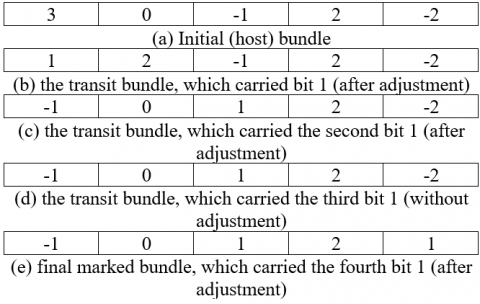Figure 4. Scenarios of embedding four data bits 1111 in the host bundle of size 5

Figures 2-4 present the examples of bit embedding in the host bundles with the size of 3-5, respectively, that was performed using our proposed method with t = 9. Figure 2(a) illustrates the initial (host) bundle, Figure 2(b) presents the transit bundle, which carried data bit “1” without adjustment (according to Steps 2 and 3 of Algorithm 1), and Figure 2(c) presents the final form of the bundle (marked bundle), which carried another data bit “1” after adjustment. The figure displays the aligned values as three numbers surrounded by squares. Similarly, according to Steps 8–10 of Algorithm 1, Figures 3 and 4 illustrate the scenarios of embedding data bits in a host bundle of size 4 and 5. Furthermore, the mean absolute error (MAE) computed from Figures 2(a) and 2(c), Figures 3(a) and 3(d), and Figures 4(a) and 4(e) were 1, 1, and 1.8, respectively. We assumed that no error (or data corruption) occurred during transmission at the receiver site. According to Steps 2–5 of Algorithm 2, data bits “11” could be extracted using the marked bundle illustrated in Figure 2(c). Similarly, both the hidden bits “111” and “1111” could be extracted using the marked bundles illustrated in Figures 3(d) and 4(e), respectively, which is in accordance with Steps 6–8 of Algorithm 2.

From the above two algorithms we can see that the computation complexity of the proposed decision rule and coefficient adjustment is quite simple. For example, a host bundle whose size is larger than 2, it requires the computation of (n-2) ´ (n-1) ´ addition, (n-2) ´ division, subtraction, and absolute value; if the size of a host bundle is 2, it only needs the computation of subtraction and absolute value. Furthermore, the process of coefficient calibration just uses increment and decrement to achieve the goal within a finite number of times. That is, the adjustment values would be limited between -t and t. It may take a little computation time at the Steps 3-4 and Steps 7-8 of Algorithm 1 during coefficient adjustment procedure. A possible way of reducing computation time is not use iterative adjustment, that is, we could directly force and chane the values of the target coefficients to be satisfied with decision criteria. It would result in a lower SNR value obtained by our method. That is the reason why we employ simple decision and (iterative) coefficient calibration in our proposed method. To obtain extreme hiding storage (and if one neglects perceived quality), the optimal payload of the proposed method with host bundleof size $n$ and without violation was $\frac{n-1}{n} \times|\Psi| .$ By contrast, to obtain high perceived quality, data bits can only be embedded in the $I_{H}$ sub-band of IWT coefficients. Because the distortion caused by the proposed method by embedding secret bits in $I_{H}$ was considerably less than that caused by the proposed method by embedding secret bits in $I_{L} .$ However, in this case, the payload without violation would be at most $\frac{2}{3} \times\left|I_{L}\right|$ when the size of a host bundle was $3 .$ In addition, the value of $\tau$ was not necessarily constant. When $\tau$ value was small, SNR increased and vice versa. Moreover, when the size of host bundles was large, a large payload was obtained using the proposed method. Furthermore, for a given constant value of $\tau$, the maximum payload of the proposed method with a certain size of host bundle can be observed in the ECG host. The proposed method with a large $\tau$ provides more robust performance than it provides with any smaller $\tau$. Figure 5 presents the block diagram of the proposed method.

## 5.pngFigure 5. Block diagram of the proposed method

3. Experimental Results

Demonstrations of the proposed method by using an Intel(R) Core(TM) i5 1.7 GHz Laptop with 4 GB RAM. The average central processing unit (CPU) time for our method was approximately 0.0310 s. Several ECG host signals obtained from the MIT-BIH arrhythmia database  were used as test data, and each ECG host consisted of 30,000 samples. Both the control integer t and size of a host bundle were not constant during simulations. Three objective measurements, namely, SNR, MAE, and PRD were used for performance evaluation. They are defined as follows:

$S N R=10 \log _{10} \frac{\sum_{i} s_{i}^{2}}{\sum_{i}\left(s_{i}-\hat{s}_{i}\right)^{2}}$     (2)

$M A E=\frac{1}{N} \sum_{i=1}^{N}\left|s_{i}-\hat{s}_{i}\right|$     (3)

and

$P R D=\sqrt{\frac{\sum\left(s_{i}-\hat{s}_{i}\right)^{2}}{\sum_{i} s_{i}^{2}}}$    (4)

where, $s_{i}$ and $\hat{s}_{i}$ are the coefficients in original ECG and marked ECG, respectively. The resultant SNR, MAE, and payload for the proposed method with three distinct values of $\tau$ are listed in Tables 1-3, respectively. In our method, the smaller value of $\tau$ caused the value of SNR to become larger, and a low payload was obtained. The average SNRs, MAEs, and payloads of the proposed method with $\tau=9 / 22 / 55$ were 49.90/45.14/40.31 dB, 1.9335/3.0780/4.7157, and 21,704/24,313/25,806 bits, respectively. In addition, the relationship between payload and SNR of the proposed method that was obtained using host bundles of various sizes is presented in Figure 6. Of the six ECG signals that were tested as host bundles, ECG 100 exhibited the most efficient performance, followed by ECG 102, ECG 101, ECG 232, and ECG213. The performance of ECG 116 ranked the last place, which means ECG116 providing less SNR and hiding-capacity than other five tests. This can be confirmed from Tables 1-3. The resultant SNR of ECG116 always below the average SNR value with 1-2 dB. The average SNRs of these six tests were 70, 60, and 50 dB when their payload sizes were 3.1, 9.7, and 21.2 kb, respectively. Figure 7 presents the trade-off between payload, SNR, and the size of host bundle for the proposed method. The larger the bundle size is, the larger the payload is, and the lower the SNR is, and vice versa. Similar performance, as shown in Figure 8, was demonstrated when the z-axis was replaced by various values of $\tau$. As specified in the last paragraph of Sec. 2.2, the larger values of $\tau$, the better robustness performance obtained by the proposed method. Furthermore, close observations obtained (within the first 3 s with $\tau$=9) from the marked ECGs are presented in Figure 9. The marked signal introduced by our methods (red line) was approximately similar to the original one (blue line). This implies that the distortion caused by our method was not significant. namely, the resultant perceived quality is not bad. The performance comparison between various methods is presented in Table 4. Both the average SNR and payload of the proposed method are the best among the compared methods. A difference image generated from Figure 9 is illustrated in Figure 10. The disturbances in the lines that appeared in Figures 10(a)-10(e) were not so drastic, indicating they exhibited more favorable performance than the Figure 10(f) did. Moreover, Figure 11 indicates that both the SNR and payload performance of the proposed method were superior to those of Pandey et al.  and Yang and Wang . Especially, both the SNR and hiding capacity of our method is significant better than other two techniques as payload size being increased to 20 kb.

Table 1. SNR/MAE/payload performance of the proposed method with $\tau$=9

 ECG data SNR/MAE/payload Bundle size 100 50.18/1.9652/24,146 15 101 50.27/1.9028/22,427 9 102 50.04/1.9828/23,542 11 103 49.93/1.9648/21,473 10 104 49.95/1.9726/21,312 10 105 50.49/1.9001/21,195 9 106 50.14/1.9685/20,911 10 107 52.26/1.4144/16,637 11 108 49.35/2.1747/23,154 14 109 50.92/1.6808/19,532 10 111 50.28/1.9607/21,651 10 112 48.44/2.0797/22,119 10 113 50.95/1.8418/21,844 10 114 49.85/2.1248/24,335 12 115 49.57/1.9234/23,364 12 116 48.97/1.8189/19,044 9 117 48.87/2.0211/22,493 10 118 48.89/1.8517/18,195 9 119 49.51/1.7968/20,466 10 121 49.07/1.9788/24,427 10 122 48.36/2.0692/22,099 10 123 48.88/2.0263/22,734 11 124 49.08/1.9193/23,079 12 200 50.40/1.8750/20,474 9 201 50.40/1.9099/23,160 9 202 50.46/2.0106/23,599 12 203 50.36/1.8143/17,571 10 205 49.37/2.0682/23,488 10 207 51.08/1.7824/21,992 10 208 50.66/1.8318/18,876 8 209 50.33/1.9125/21,139 7 210 50.27/1.9896/23,208 10 232 49.08/2.2722/22,542 15 Average 49.90/1.9335/21,704 -

Table 2. SNR/MAE/payload performance of the proposed method with $\tau$=22

 ECG data SNR/MAE/payload Bundle size 100 45.41/2.9671/26,029 15 101 45.04/3.0394/24,897 9 102 45.95/2.9163/25,577 11 103 44.89/3.2131/24,149 10 104 45.03/3.2777/24,099 10 105 45.83/2.9305/23,977 9 106 44.71/3.3543/24,099 10 107 45.87/2.7020/19,675 11 108 44.59/3.5170/26,027 14 109 45.70/2.8330/23,000 10 111 45.53/3.1057/24,749 10 112 43.80/3.3185/24,861 10 113 46.61/2.6667/23,961 10 114 46.28/2.9440/26,167 12 115 44.78/3.0021/25,208 12 116 43.52/3.2029/22,300 9 117 44.75/2.9114/24,801 10 118 42.95/3.4706/23,140 9 119 44.25/2.9620/23,055 10 121 45.62/2.7462/25,829 10 122 43.48/3.4689/23,740 10 123 44.71/2.9447/24,829 11 124 44.47/2.9404/25,151 12 200 45.44/3.1493/23,531 9 201 46.65/2.7663/24,927 9 202 46.21/2.9139/25,577 12 203 44.69/3.4782/21,941 10 205 45.60/3.0624/25,240 10 207 45.70/2.9746/24,655 10 208 44.64/3.4608/22,807 8 209 46.13/2.8485/23,450 7 210 46.81/2.7528/25,056 10 232 44.11/3.7972/25,813 11 Average 45.14/3.0780/24,313 -

Table 3. SNR/MAE/payload performance of the proposed method with $\tau$=55

 ECG data SNR/MAE/payload Bundle size 100 40.89/4.2992/26,943 15 101 41.59/4.2326/25,809 9 102 40.60/4.3088/26,524 11 103 39.81/5.1745/25,678 10 104 39.25/5.2619/25,769 10 105 40.17/4.7398/25,475 9 106 39.59/5.3807/25,811 10 107 37.53/6.5479/23,297 11 108 39.36/5.4429/27,317 14 109 38.98/5.3496/25,305 10 111 40.29/4.8932/26,246 10 112 40.22/4.4310/26,213 10 113 40.86/4.1979/25,537 10 114 41.49/4.1508/27,243 12 115 41.05/4.1554/26,227 12 116 37.92/5.4749/24,436 9 117 39.52/4.4712/26,212 10 118 38.69/5.2909/25,001 9 119 37.77/5.5064/25,390 10 121 42.16/3.3453/26,324 10 122 38.54/5.5784/25,566 10 123 39.02/4.8692/26,201 11 124 40.37/4.2905/26,426 12 200 40.77/4.6289/25,327 9 201 43.32/3.3566/25,726 9 202 41.37/4.1713/26,661 12 203 38.91/6.1755/25,001 10 205 42.86/3.7525/25,968 10 207 40.60/4.4125/26,003 10 208 39.96/5.5244/24,702 8 209 43.13/3.6387/24,467 7 210 42.51/3.6497/25,978 10 232 40.99/4.9134/26,830 11 Average 40.31/4.7157/25,806 -

Table 4. SNR/net-payload (bits) performance comparison between various methods

 ECG data set Yang and  Lin  Yang and  Wang  Yang and  Wang  Our method 100 42.37/15,000 44.15/20,000 48.19/19,604 50.18/24,146 101 42.54/15,000 44.09/20,000 47.39/19,610 50.27/22,427 102 44.44/15,000 45.44/20,000 51.60/19,602 50.04/23,542 103 38.96/15,000 40.86/20,000 44.92/19,600 49.93/21,473 104 42.06/15,000 42.74/20,000 46.84/19,602 49.95/21,312 105 44.05/15,000 45.71/20,000 47.66/19,602 50.49/21,195 111 46.76/15,000 48.22/20,000 49.43/19,630 50.28/21,651 112 44.86/15,000 46.59/20,000 48.32/19,658 48.44/22,119 113 38.49/15,000 40.31/20,000 46.23/19,602 50.95/21,844 114 48.05/15,000 49.09/20,000 51.59/19,604 49.85/24,335 115 37.99/15,000 39.77/20,000 44.51/19,608 49.57/23,364 121 48.40/15,000 49.91/20,000 51.97/19,602 49.07/24,427 122 40.53/15,000 42.30/20,000 44.69/19,602 48.36/22,099 123 39.13/15,000 40.79/20,000 46.49/19,602 48.88/22,734 124 41.93/15,000 43.54/20,000 46.36/19,602 49.08/23,079 200 42.18/15,000 43.98/20,000 47.36/19,608 50.40/20,474 201 46.14/15,000 47.62/20,000 50.13/19,612 50.40/23,160 202 46.33/15,000 47.82/20,000 50.16/19,632 50.46/23,599 203 40.64/15,000 42.13/20,000 43.27/19,622 50.36/17,571 232 46.96/15,000 48.65/20,000 51.58/19,620 49.08/22,542 Average 43.14/15,000 44.69/20,000 47.93/19,611 49.80/22,355

## 6.png## 7.pngFigure 7. Relationship between payload, SNR, and bundle size of the proposed method with $\tau$=9

## 8.png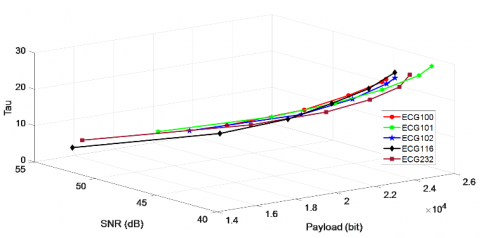Figure 8. Relationship between payload, SNR, and $\tau$ of the proposed method with the bundle of size 15

## 91.png## 92.png## 93.pngFigure 9. Close observations of the marked ECG generated using the proposed method with t = 9

## 101.png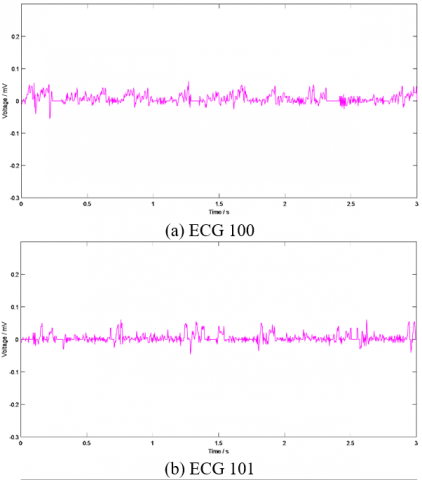## 102.png## 103.pngFigure 10. Difference images generated from the close observations of the marked ECG generated using the proposed method

## 11.pngFigure 11. Performance comparison between various methods

Two examples of survived watermarks after the marked ECG 100 (with $\tau$=22) was manipulated are presented in Tables 5 and 6. The size of a host bundle was 15. Two different input binary images of size $161 \times 161$ were used as an input watermark. The resultant SNR of the marked ECG 100 was approximately 45 dB. Notably, the value of PRD = 0 when the marked ECGs were not being manipulated. The 2nd and 3rd rows of the Table 5 indicate that the watermarks extracted from the mark ECG attacked using the additive white Gaussian noise (AWGN) with 0.1 and 1 dB were recognized. Similarly, the extracted watermark presented in the 4th row of Table 5 that survived the cropping attack (with 33% off) was identified. Additionally, the extracted watermark (in the 8th row of Table 5) was identified when the last three bits of the marked samples were truncated. Although the PRD value of survived watermarks that were manipulated using inversion was close to 1, it was recognized. Our method exhibited robust performance against translation (+1500, −1500) and scaling (*0.9, *1.2) attacks. Table 6 indicates similar robustness generated by our method. Furthermore, the PRD performance of the proposed method under various attacks such as AWGN, scaling, and translation with different factors is presented in Figure 12. Figure 12(a) indicates that the tolerance test factor of our method for AWGN attack was 0.1 dB. the higher the SNR is, the lower the PRD is; thus, increasing SNR results in a good perceived quality. Figure 12(b) indicates the range attacked by scaling was from 0.9 to 1.2. Figure 12(c) indicates that our method resisted translation attacks in the range from −2000 to +2000. Finally, from Tables 5-6 and Figure 12, we concluded that the proposed method is capable of resisting various types of attacks.

Table 5. Examples of survived watermarks from the manipulations of marked ECG 100

Attacks

Survived Watermarks

Null-attack PRD = 0.0000

## 551.jpg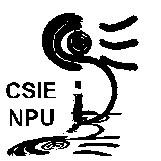AWGN (with SNR 0.1 dB) PRD = 0.6845

## 552.jpgAWGN (with SNR 1 dB) PRD = 0.6564

## 553.jpgCropping (33% off) PRD = 0.8295

## 554.jpg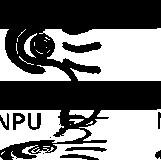Inversion PRD = 0.9879

## 555.jpg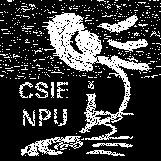Scaling (*0.9) PRD = 0.6689

## 556.jpg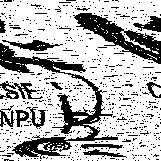Scaling (*1.2) PRD = 0.6570

## 557.jpg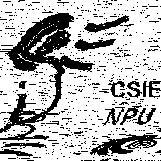Truncation+ PRD = 0.8018

## 558.jpg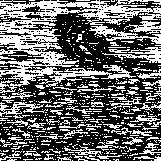Translation (+1500) PRD = 0.0000

## 559.jpgTranslation (-1500) PRD = 0.6828

## 510.jpg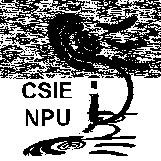+The last three bits of the marked data were truncated.

Table 6. Another example of survived watermarks from the manipulations of marked ECG 100

Attacks

Survived Watermarks

Null-attack PRD = 0.0000

## 661.jpg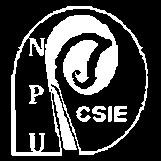AWGN (with SNR 0.1 dB) PRD = 1.1523

## 662.jpgAWGN (with SNR 1 dB) PRD = 1.1287

## 663.jpgCropping (33% off) PRD = 1.0905

## 664.jpgInversion PRD = 1.5630

## 665.jpg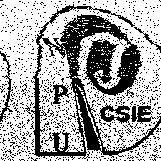Scaling (*0.9) PRD = 1.1797

## 666.jpg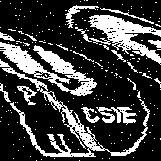Scaling (*1.2) PRD = 1.2158

## 667.jpgTruncation+ PRD = 1.1671

## 668.jpgTranslation (+1500) PRD = 0.0000

## 669.jpgTranslation (-1500) PRD = 1.1131

## 6610.jpg+The last two bits of the marked data were truncated

## 12.png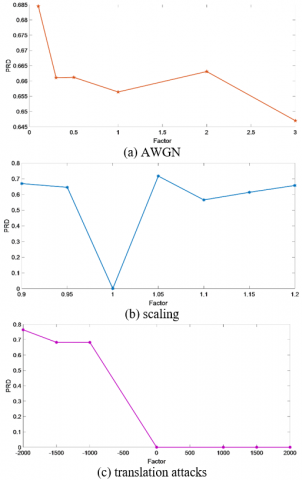Figure 12. PRD performance obtained using the proposed method against three types of attacks under various test factors

4. Conclusions

In this study, we established a cumulative data hiding approach in ECG signals based on a 1D IWT domain. According to the predetermined criterion with host bundles of various sizes, sensitive data can be successfully embedded in the IWT coefficients of ECG hosts. Experimental results confirmed that the average SNR and payload of our proposed method are superior to those of the conventional ECG steganography. Moreover, the proposed method exhibited robust performance, which has rarely been observed in existing ECG steganography techniques. The proposed method exhibited the merits of high hiding capacity and superior resultant perceived quality. Moreover, our method was resistant toward attacks such as cropping, inversion, scaling, translation, truncation, and Gaussian noise addition. Because the procedures of bit embedding and extraction are simple and the computation time is short, our method can be applied in mobile biometric devices.

Nomenclature
 $I_{L}$ low subband coefficients of IWT $I_{H}$ high subband coefficients of IWT $\Theta$ $\Psi$ decision criterion host ECG data $\hat{\Psi}$ Marked ECG data Greek symbols $\tau$ control parameter $S k$ the kth element of the host ECG data $\phi$ offset $\gamma$ offset $\beta$ offset
References

 Cox, I.J., Miller, M.L. Bloom, J.A., Fridrich, J., Kalker, T. (2008). Digital Watermarking and Steganography. 2nd Ed. Morgan Kaufmann, MA, USA.

 Raggo, M., Hosmer, C. (2013). Data hiding: Exposing concealed data in multimedia, operating systems. Mobile Devices and Network Protocols, Syngress, MA, USA.

 Eielinska, E., Mazurczyk, W., Szczypiorski, K. (2014). Trends in steganography. Communications of the ACM, 57(3): 86-95. https://doi.org/10.1145/2566590.2566610

 Liu, S., Pan, Z., Song, H. (2017). Digital image watermarking method based on DCT and fractal encoding. IET Image Proc., 11(10): 815-821. https://doi.org/10.1049/iet-ipr.2016.0862

 Hsiao, C.Y., Tsai, M.F., Yang, C.Y. (2018). Simple and robust watermarking scheme based on square-root-modulus technique. Multimedia Tools and Applications, 77(23): 30419-30435. https://doi.org/10.1007/s11042-018-6121-3

 Kozat, S.S., Vlachos, M., Lucchese, C., Herle, H.V., Yu, P.S. (2009). Embedding and retrieving private metadata in electrocardiograms. Journal of Medical Systems, 33(4): 241-259. https://doi.org/10.1007/s10916-008-9185-1

 Ibaida A., Khalil, I.  (2013). Wavelet-based ECG steganography for protecting patient confidential information in point-of-care systems. IEEE Transactions on Biomedical Engineering, 60(12): 3322-3330. https://doi.org/10.1109/TBME.2013.2264539

 Chen, S.T., Guo, Y.J., Huang, H.N., Kung, W.M., Tseng, K.K., Tu, S.Y. (2014). Hiding patients confidential data in the ECG signal via transform-domain quatization scheme. Journal of Medical Systems, 38(6): 54. https://doi.org/10.1007/s10916-014-0054-9

 Jero, S.E., Ramu, P., Ramakrishnan, S. (2014). Discrete wavelet transform and singular value decomposition based ECG steganography for secured patient information transmission. Journal of Medical Systems, 38(10): 132. https://doi.org/10.1007/s10916-014-0132-z

 Jero, S.E., Ramu, P. (2016). Curvelets-based ECG steganography for data security. Electronics Letters, 52(4): 283-285. https://doi.org/10.1049/el.2015.3218

 Yang, C.Y., Lin, K.T. (2016). Hiding data in electrocardiogram based on IWT domain via simple coefficient adjustment. The 4th Int. Conf. on Annual Conference on Engineering and Information Technology, March 29-31, Kyoto, Japan.

 Yang, C.Y., Wang, W.F. (2016). Effective electrocardiogram steganography based on coefficient alignment. Journal of Medical Systems, 40(3): 66. https://doi.org/10.1007/s10916-015-0426-9

 Jero, S.E., Ramu, P., Ramakrishnan, S. (2016). Imperceptibility-robustness tradeoff studies for ECG steganography using continuous ant colony optimization. Expert Systems with Applications, 49: 123-135. https://doi.org/ 10.1016/j.eswa.2015.12.010

 Pandey, A., Saini, B.S., Sood, N. (2017). An integrated approach using chaotic map & sample value difference method for electrocardiogram steganography and OFDM based secured patient information transmission. Journal of Medical Systems, 41(12): 187. https://doi.org/10.1007/s10916-017-0830-4

 Yang, C.Y., Wang, W.F. (2017). High-capacity ECG steganography with smart offset coefficients. The 13th International Conference on Intelligent Information Hiding and Multimedia Signal Processing, 81: 12-15. https://doi.org/10.1007/978-3-319-63856-0_16

 Yang, C.Y., Wang, W.F. (2018). An improved high-capacity ECG steganography with smart offset coefficients. International Conference on Intelligent Information Hiding and Multimedia Signal Processing, 110: 3-10. https://doi.org/10.1007/978-3-030-03748-2_1

 Calderbank, A.R., Daubechies, I., Sweldens, W., Yeo, B.L. (1998). Wavelet transforms that map integers to integers. Applied and Computational Harmonic Analysis, 5(3): 332-369. https://doi.org/10.1006/acha.1997.0238

 Moody, G.B., Mark, R.G. (2001). The impact of the MIT-BIH arrhythmia database. IEEE Engineering in Medicine and Biology Magazine, 20(3): 45-50. https://doi.org/10.1109/51.932724